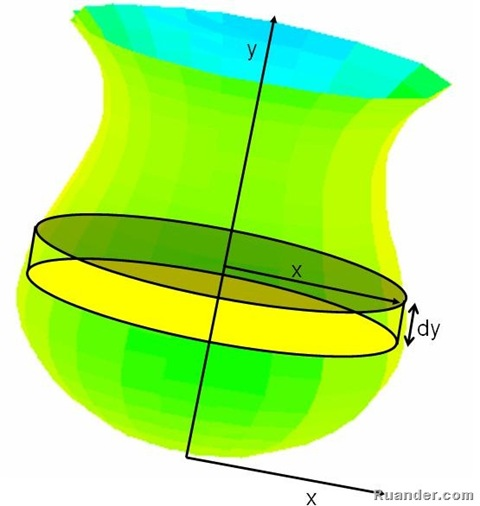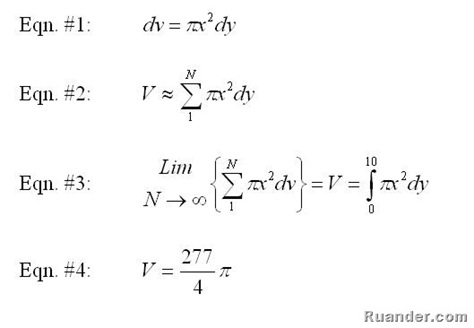## Pages

### Finding Volumes Using Differential Disks

A few days ago I posted a comment on Solids of Revolution. Days later I was chatting with an engineer friend of mine in Costa Rica. He suggested to me posting the solution procedure to find the volume of the vase, so here it is.Start with a small differential disk of radius x and thickness dy (as shown in the picture). Find the differential volume of the disk using equation #1. Create N number of disks along the height of the vase from y=0 to y=10 and add all the differential volumes together to estimate the total volume of the vase as equation #2 shows. Taking the limit as N tends to infinity (dv→0) we get equation #3. Plug in the value for x in terms of y inside the integral (x=0.0437y3-0.7125y2+3.15y+4 UNITS IN INCHES).Evaluating the integral we get that the volume is approximately 217.56 in3 (Equation #4). This is about 3.56 liters or about 10 cans of pop. Not bad!